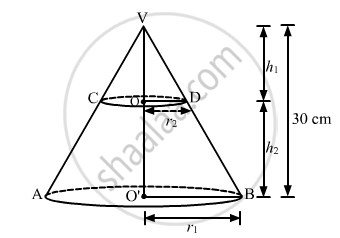Advertisement Remove all ads

# The Height of a Cone is 30 Cm. a Small Cone is Cut off at the Top by a Plane Parallel to the Base. If Its Volume Be 1 27 of the Volume of the Given Cone, Then the Height Above the - Mathematics

MCQ

The height of a cone is 30 cm. A small cone is cut off at the top by a plane parallel to the base. If its volume be $\frac{1}{27}$ of the volume of the given cone, then the height above the base at which the section has been made, is

#### Options

• 10 cm

• 15 cm

• 20 cm

• 25 cm

Advertisement Remove all ads

#### SolutionLet VAB be cone of height 30 cm and base radius r1 cm.

Suppose it is cut off by a plane parallel to the base at a height h2 from the base of the cone.

Clearly

$∆ VOD~ ∆ VO'B$

Therefore,

$\frac{OV}{O'V} = \frac{OD}{O'B}$

$\Rightarrow \frac{h_1}{30} = \frac{r_2}{r_1}$

But,

$\text { Volume of cone VCD} = \frac{1}{27}\text { Volume of cone VAB }$

$\Rightarrow \frac{1}{3}\pi \left( r_2 \right)^2 h_1 = \frac{1}{27}\left( \frac{1}{3}\pi \left( r_1 \right)^2 30 \right)$

$\Rightarrow \left( \frac{r_2}{r_1} \right)^2 h_1 = \frac{10}{9}$

$\Rightarrow \left( \frac{h_1}{30} \right)^2 h_1 = \frac{10}{9}$

$\Rightarrow h_1 = 10$

Hence,

Required height

= 30 -10

= 20 cm

Is there an error in this question or solution?
Advertisement Remove all ads

#### APPEARS IN

RD Sharma Class 10 Maths
Chapter 14 Surface Areas and Volumes
Q 11 | Page 88
Advertisement Remove all ads
Advertisement Remove all ads
Share
Notifications

View all notifications

Forgot password?